Courses

# Mathematics Test 10 - Trigonometry

## 30 Questions MCQ Test JEE Main Mock Test Series 2020 & Previous Year Papers | Mathematics Test 10 - Trigonometry

Description
This mock test of Mathematics Test 10 - Trigonometry for JEE helps you for every JEE entrance exam. This contains 30 Multiple Choice Questions for JEE Mathematics Test 10 - Trigonometry (mcq) to study with solutions a complete question bank. The solved questions answers in this Mathematics Test 10 - Trigonometry quiz give you a good mix of easy questions and tough questions. JEE students definitely take this Mathematics Test 10 - Trigonometry exercise for a better result in the exam. You can find other Mathematics Test 10 - Trigonometry extra questions, long questions & short questions for JEE on EduRev as well by searching above.
QUESTION: 1

### If m= sin6x + cos6x, then m belongs to the interval

Solution:
m=sin^6x+cos^6x=(sin^2x+cos^2x)(sin4x+cos^4x-sin^2xcos^2x)

as a^3+b^3=(a+b)(a^2-ab+b^2)

we know that,

sin^2x+cos^2x=1

m=((sin^2x+cos^2x)^2-3sin^2xcos^2x)

m=1-3sin^2xcos^2x

m=1-3/4(sin2x)^2

we know 0<=sin^2A<=1,

so -1<=-sin^2 2x<=0 multiply with -1,

-3/4<=-3/4(sin^2 2x)<=0 multiply with 3/4,

1/4<=m<=1

therefore "m" belongs to[1,1/4].
QUESTION: 2

Solution:
QUESTION: 3

### if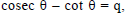then the value of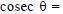Solution:
QUESTION: 4

if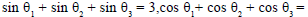Solution:
QUESTION: 5
In a triangle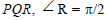.If tan (P/2) and than (Q/2) are the roots of the equation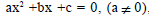then
Solution:
QUESTION: 6
If m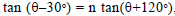then (m n)/(m–n) =
Solution:
QUESTION: 7

Minimum value of 3cosx + 4sinx +5?

Solution:
QUESTION: 8
The expression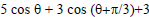) lies between
Solution: 5 cos a+3 cos (a + π3)+3 ⇒ 5 cos a + 3 [  cos(π3)cos a - sin (π3) sin a ]+ 3 As we know ( cos ( a + b ) = cos a cos b - sin a sin b ) ⇒5 cos a + 3(12)cos a - 3(32) sin a+3 since cos(π3) = 12, sin(π3) = 32 ⇒132 cos a - 3(32 ) sin a + 3 a cos a + b sin a + c       minimum value is  c-a2 + b2 ⇒132 cos a - 3(32 ) sin a + 3 minimum value   3- 13222 +33222 ⇒ 3 - 1694 + 274 ⇒ 3 - 49 ⇒ 3 -  7 ⇒ -4 maximum value is  3 + 13222 +33222  ⇒ 3 + 1694 + 274 ⇒ 3 + 7 ⇒ 10
QUESTION: 9

The maximum value of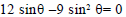is

Solution:
QUESTION: 10

if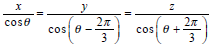then x+y+z =

Solution:
QUESTION: 11

The value of expression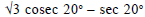is equal to

Solution:
QUESTION: 12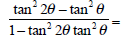Solution:
QUESTION: 13
If the angle A of a triangle ABC is given by the equation 5 cos A 3 = 0, then sin A and tan A are the roots of the equation
Solution:
QUESTION: 14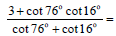Solution:
QUESTION: 15

If A B =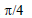then (tanA + 1)(tanB + 1) is equal to

Solution:
QUESTION: 16
For a positive integer n,let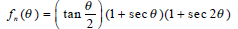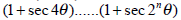,Then
Solution:
QUESTION: 17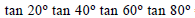=
Solution:
QUESTION: 18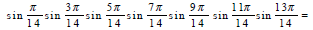Solution:
QUESTION: 19
If q =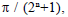then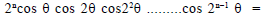Solution:
QUESTION: 20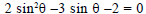the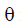=
Solution:
QUESTION: 21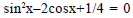Solution:
QUESTION: 22
The number of solutions of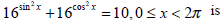Solution:
QUESTION: 23
The maximum value of sin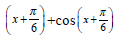in the interval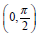is attained at
Solution:
QUESTION: 24

The equation asinx + bcosx = c where |c|>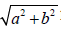has

Solution:
QUESTION: 25
If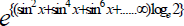satisfying the equation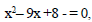then value of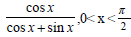is
Solution:
QUESTION: 26
The equation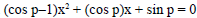,where x is a variable has real roots. Then the interval of p may be any of the following
Solution:
QUESTION: 27
The number of solutions of the equation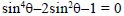which lie between 0 and 2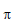is
Solution:
QUESTION: 28

Simplify 15ax2 ⁄ 5x

Solution:
QUESTION: 29
if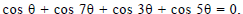then=
Solution:
QUESTION: 30
If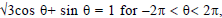then=
Solution: √3cosx+sinx=1=>√3/2cosx+1/2sinx=1/2=>(cosa)(cosx)+(sina)(sinx)=1/2(( let √3/2 be cosa and 1/2 be sina) =>cosπ/6cosx+sinπ/6sinx=1/2 =>cos( π/6-x)=1/2 =>cos(x-π/6)=cosπ/3 =>x-π/6=2nπ+/-π/3 =>x=2nπ+π/2 or x=2nπ-π/6 => here we can have all the values given in option.